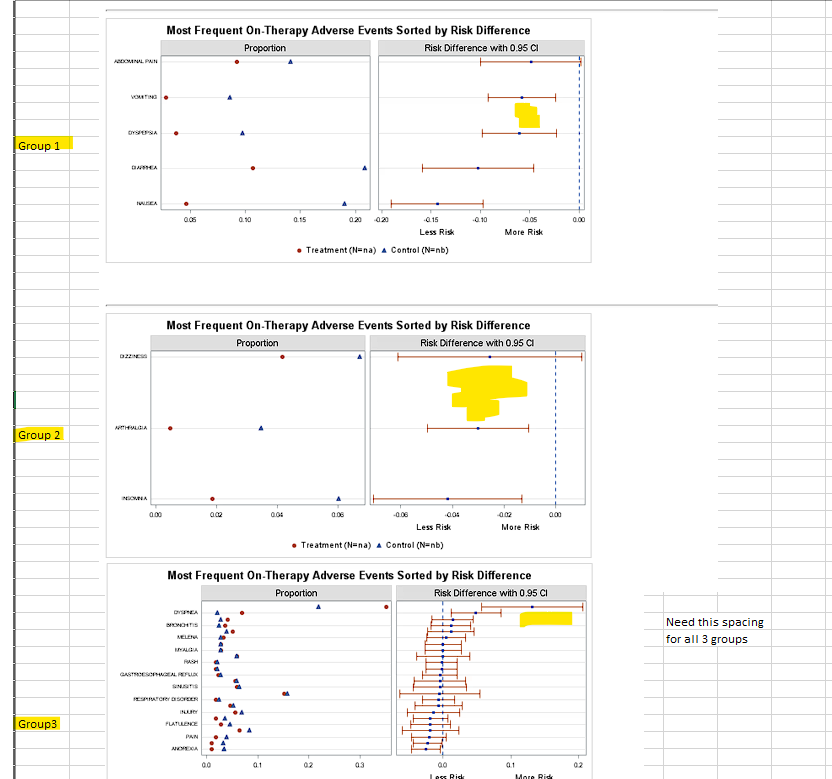## How to get uniform spacing across pages for dot plot (scatter plot) ?

Hi All,

I have a dot plot with 3 pages and grouped data.

I want to get uniform spacing for all 3 pages with space as in Group 3 below.

For ex. for Page 2, all 3 data lines should be at top with spacing of about 0.5 cm as in Group3.

Is there any option to do this ?

Thanks.``````%let gpath='C:/';
%let dpi=200;
%let na=na;
%let nb=nb;

data ae;
input Pref \$1-30 NA NB SNA SNB;
datalines;
ABDOMINAL PAIN                20    61    216    431
ANOREXIA                       2    15    216    431
ARTHRALGIA                     1    15    216    431
BACK PAIN                     10    23    216    431
BRONCHITIS                     8    11    216    431
CHEST PAIN                     9    12    216    431
CHRONIC OBSTRUCTIVE AIRWAY    76    95    216    431
COUGHING                      13    26    216    431
DIARRHEA                      23    90    216    431
DIZZINESS                      9    29    216    431
DYSPEPSIA                      8    42    216    431
DYSPNEA                       15     9    216    431
FATIGUE                        4    16    216    431
FLATULENCE                     6    20    216    431
GASTROESOPHAGEAL REFLUX        5    12    216    431
HEMATURIA                      2    14    216    431
HYPERKALEMIA                   4     9    216    431
INFECTION VIRAL               12    26    216    431
INJURY                        12    30    216    431
INSOMNIA                       4    26    216    431
MELENA                         7    12    216    431
MYALGIA                        6    12    216    431
NAUSEA                        10    82    216    431
PAIN                           4    17    216    431
RASH                           4     9    216    431
RESPIRATORY DISORDER           4    11    216    431
RHINITIS                      11    17    216    431
SINUSITIS                     13    28    216    431
UPPER RESP TRACT INFECTION    33    68    216    431
URINARY TRACT INFECTION        6    12    216    431
VOMITING                       6    37    216    431
WEIGHT DECREASE                2     9    216
run;

/*--Compute Proportions for treatment A & B, Mean and Risk--*/
data ae_risk;
set ae;
keep pref a b mean lcl ucl;
a=na/sna;
b=nb/snb;
factor=1.96*sqrt(a*(1-a)/sna + b*(1-b)/snb);
lcl=a-b+factor;
ucl=a-b-factor;
mean=0.5*(lcl+ucl);
run;

/*--Sort by mean value--*/
proc sort data=ae_risk out=ae_sort;
by mean;
run;

data ae_ref;
set ae_sort;
if mod(_n_, 2) eq 0 then ref=pref;
if _n_ <=5 then group=1 ;
else if _n_ <=8 then group=2;
else group=3 ;
run;
proc print;run;

/*--Create template for AE graph--*/
proc template;
define statgraph AEbyRelativeRisk;
dynamic _thk _grid;
begingraph;
entrytitle 'Most Frequent On-Therapy Adverse Events Sorted by Risk Difference';
layout lattice / columns=2 rowdatarange=union columngutter=5;

/*--Row block to get common external row axes--*/
rowaxes;
rowaxis / griddisplay=_grid display=(tickvalues) tickvalueattrs=(size=5);
endrowaxes;

layout overlay / border=true backgroundcolor=cxdfdfdf opaque=true;
entry "Proportion";
endlayout;
layout overlay / border=true backgroundcolor=cxdfdfdf opaque=true;
entry "Risk Difference with 0.95 CI";
endlayout;

/*--Left side cell with proportional values--*/
layout overlay / xaxisopts=(display=(ticks tickvalues)  tickvalueattrs=(size=7));
referenceline y=ref / lineattrs=(thickness=_thk) datatransparency=0.9;
scatterplot y=pref x=a / markerattrs=graphdata2(symbol=circlefilled)
name='a' legendlabel="Treatment (N=&NA)";
scatterplot y=pref x=b / markerattrs=graphdata1(symbol=trianglefilled)
name='b' legendlabel="Control (N=&NB)";
endlayout;

/*--Right side cell with Relative Risk values--*/
layout overlay / xaxisopts=(label='Less Risk                    More Risk'
labelattrs=(size=8)  tickvalueattrs=(size=7));
referenceline y=ref / lineattrs=(thickness=_thk) datatransparency=0.9;
scatterplot y=pref x=mean / xerrorlower=lcl xerrorupper=ucl
markerattrs=(symbol=circlefilled size=5);
endlayout;

/*--Centered side bar for legend--*/
sidebar / spacefill=false;
discretelegend 'a' 'b' / border=false;
endsidebar;
endlayout;
endgraph;
end;
run;

ods html close;
ods listing gpath=&gpath image_dpi=&dpi style=listing;

/*--Render the graph with grid lines without horizontal bands--*/
ods graphics / reset width=6in height=4in imagename='AEbyRelativeRisk';
proc sgrender data=ae_ref template=AEbyRelativeRisk;
dynamic _thk='0' _grid='on';
by group ;
run;

``````

4 REPLIES 4

## Re: How to get uniform spacing across pages for dot plot (scatter plot) ?

What changes are you willing to make to the yaxis labels? Since the space is changing because of label length that would be a place to start.

## Re: How to get uniform spacing across pages for dot plot (scatter plot) ?

Can change yaxis labels if display is correct.

I tried this.

Group 3 has more number of obs (24) hence getting good space.

can something like this be done ?

``````%macro graph;
%do group =1 %to 3;
data   ae_ref2 ;
set   ae_ref;
where group= &group  ;
run;

title5 Group: &group ;
proc sgrender data=ae_ref2 template=AEbyRelativeRisk;
dynamic _thk='0' _grid='on';
/*by group ;*/
run;

%end;

%mend graph;
%graph;

``````

## Re: How to get uniform spacing across pages for dot plot (scatter plot) ?

One thing might be a custom format that displays closer to the same number of characters for each value.

Add one or more *, or other special character, in the value to break text using the ROWAXIS option TICKVALUESPLITCHAR.

If the value of the text to display were "Something with*an asterisk" and the Rowaxis has Tickvaluesplitchar='*' the line would attempt to split into

Something with

an asterisk

as the label for the tick mark.

You can split one line of text multiple times but you may still have issues.

Use a smaller font for the label values.DanH_sas
SAS Super FREQ

## Re: How to get uniform spacing across pages for dot plot (scatter plot) ?

If this is a one-off kind of graphing situation, you can increase the OFFSETMIN on the ROWAXIS statement for each group run to achieve the same spacing between categories.  Hope this helps!

Discussion stats
• 4 replies
• 290 views
• 0 likes
• 3 in conversation